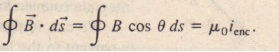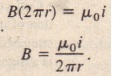# Ampere’s Law

Ampere’s Law

We can find the net electric field due to any distribution of charges with the inverse square law for the differential field if (Eq. 30-2), but if the distribution is complicated,  we may have to use a computer. Recall, however. that if the istribution has   lanar. cylindrical. or spherical symmetry, we can apply Gauss’ law to find the net electric field with on siderably less effort.  Similarly, we can find the net magnetic field due to.Ell)’ distribution of currents ith the inverse-square law for the differential field dB (Eq. 30-5), but again wemay have to use a computer for a complicated distribution. However, if the distribution  has some symmetry, we may be able to apply Ampere’s law to find the agnetic field with considerably less effort. This law, which can be derived from the Biot-Savart law, has traditionally been credited to Andre Marie Ampere (1775-  1836), for whom the SI unit of current is named. However, the law actually was  advanced by English physicist James Clerk Maxwell. Ampere’s law is.

The circle on the integral sign means that the scalar (or dot) product B ‘ d’S is to be integrated around a closed loop, called an Amerindian loop. The current ienc.on the  right is the net current encircled by that loop.- To see the meaning of the scalar product B . d,~ and its integral, let us first apply Ampere’s law to the general situation of Fig. 30-11. The figure shows cross  sections of three long straight wires that carry currents il ‘ i2, and i) either directlyinto or directly out of the page. An arbitrary Amperian loop lying in the plane of the page eocircles two of the currents but not the third. The counterclockwise direction marked on the loop indicates the arbitrarily chosen direction of integration for Eq.30-16. To apply Ampere’s law, we mentally divide the loop into differential vector  elements d’S that are everywhere directed along the tangent to the loop in the directionof integration. Assume that at the location of the ele~ent d.~ shown in Fig.  30-11, the net magnetic field due to the three currents is B. Because the wires are perpendicular to the page, we know that the magnetic field at d’S due to each current.

is in the plane of thus, their net magnetic field ii at d’S must also be in that plane. However, we do not know the orientation of ii within the plane. In  ii is arbitrarily drawn at an angle () to the direction of d’S.The scalar product ii· ds on the left side of Eq. 30-16 is then equal to B cos () ds. Thus, Ampere’s law can be written as.We can now interpret the scalar product ii . d’S as being the product of a length ds of the Amerindian loop and the field component B cos () that is tangent to the loop. Then we can interpret the integration as being the summation of all such products  around the entire loop.When we can actually perform this integration, we do not need to know the direction of ii before integrating. Instead, we arbitrarily assume ii to be generally in the  direction of integration (as in Fig. 30-11). Then we use the following curled straight right-hand rule to assign a plus sign or a minus sign to each of the currents that make up the net encircled current .

Curl your right hand around the Amerindian loop, with the fingers pointing in the direction of integration. A current through the loop in the general direction of your outstretched thumb is assigned a plus sign, and a current generally in the opposite direction is assigned a minus sign.

Finally, we solve Eq. 30-17 for the magnitude of ii.If B turns out positive, then the direction we assumed for ii is correct. If it turns  out negative, we neglect the minus sign and redraw B in the opposite direction. In  we apply the curled-straight rule for Ampere’s law 10 the situation of  With the indicated counterclockwise direction of integration, the net current encircled by the loop is.

The Magnetic Field Outside a Long Straight Wire with Current

shows a long straight wire that carries current; directly out of the page. Equation 30-6 tells us that the magnetic field produced by the current has the same .

Ii has cylindrical symmetry about the wire, We can take advantage of that symmetry   simplify the integral in Ampere’s law (Eqs, 30-16 and 30-17) if we encircle the wire with a concentric circular Amerindian loop of radius r, as in  The mange LC field Ii then has the same magnitude B at every point on the loop. We shall integrate counterclockwise, so that ds has the direction shown in. , We can further simplify the quantity B cos  by noting that Ii is tangent to the loop at every point along the loop, as is ds. Thus, if and ds are either parallel or anti parallel at each point of the loop, and we shall arbitrarily assume the former. Then at every point the angle (J between d’S and if is 0°, so cos (J =cos 0° = I. The integral in  then becomes.Note  above’ is the summation of all he line segment lengths ds around the \ circular loop; that is, it simply gives the circumference 27Tf of the loop. Our right-hand rule gives us a plus sign for the current of  The right side of Ampere’s law becomes +J-Loi and we then have.With a slight change in notation, this is Eq. 30-6, which we derived earlier-with considerably more effort-using the law of  Bio and Savant. In addition, because the magnitude B turned out positive, we know that the correct direction of Ii must be the one shown in .

The Magnetic Field Inside a Long Straight wire with Current.To find the right side of Ampere’s law, we note that because the current is uniformly distributed, the current keno encircled by the loop is proportional to the area encircled by the loop; that is.Thus, inside the wire, the magnitude B of the magnetic “field is proportional to r; that magnitude is zero at the center and a maximum at the surface, where r = R. Note that Eqs. 30-19 and the same value for B at r = R; that is, the expressions for the magnetic field outside the wire and inside the wire yield the same result at the surface of the wire.

Sample Problem

Figure ,10-15a shows the cross section of a long conducting cylinder with inner radius a – 2,0 cm and outer radius b = 40 cm  The cylinder carries a current out of the page. and th; cu.re«. de”Sl y II the GLOSS section is given by J = cr2• With c = 3.0 X  106 A/m4 and, in meters. What is the magnetic field if at a point that is 3.0 er from the central axis of the cy under?

SOLUTION: The point at which we want to evaluate if i~ inside the material of the conducting cylinder. between its inner and outer radii, We note that the current distribution has cylindrical symmetry  it is the same all around the cross section for a.iy given radius). Thus. the Key Idea here IS that the symmetry allows us to use Ampere’s law to find if at the point. We first dr  w the Amerindian loops gown in  The loop is concentric with the cylinder and  has radius r = 3.0 em, because we want to evaluate if at that distance from the cylinder’s central axis. Next. we must compute the current  that is encircled by the Riparian loop. Howe er. a second Key Idea is that we cannot set up a proportionality as in  here the current’s .s net uniformly distributed. Instead. following the procedure of  we must integrate the current density tromp the chancy s .nine radius a t’J the loop radius.The direction of integration indicated in  is (arbitrarily)  clockwise. Applying the right-hand rule for Ampere’s law to that loop, we find that we should take i.n< as negative because the current is directed out of the page but our thumb is directed into the page. We next evaluate the left side of Ampere’ s law exactly as  we did in  and we again obtain Eq. 30-20, Then Ampere’slaw.